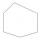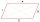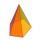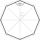# Right triangle + polygon - math problems

#### Number of problems found: 50

• Six-sided polygonIn a six-sided polygon. The first two angles are equal, the third angle is twice (the equal angles), two other angles are trice the equal angle, while the last angle is a right angle. Find the value of each angle.
• Find theFind the content of a regular 12 sided polygon, if its side a = 12 cm.
• 6 regular polygonIt is given 6 side regular polygon whose side is 5 cm. Calculate its content area. Compare how many more cm2 (square centimeters) has a circle in which is inscribed the 6-gon.
• Regular n-gonWhich regular polygon have a radius of circumscribed circle r = 10 cm and the radius of inscribed circle p = 9.962 cm?
• Center of gravityIn the isosceles triangle ABC is the ratio of the lengths of AB and the height to AB 10:12. The arm has a length of 26 cm. If the center of gravity T of triangle ABC find area of triangle ABT.
• HexagonThere is regular hexagon ABCDEF. If area of the triangle ABC is 22, what is area of the hexagon ABCDEF? I do not know how to solve it simply....
• PentagonCalculate the area of a regular pentagon, which diagonal is u=17.
• OctagonWe have a square with side 84 cm. We cut the corners to make his octagon. What will be the side of the octagon?
• DecagonCalculate the area and circumference of the regular decagon when its radius of a circle circumscribing is R = 1m
• Hexagon ACalculate area of regular hexagon inscribed in circle with radius r=9 cm.
• n-gon IIWhat is the side length of the regular 5-gon circumscribed circle of radius 11 cm?
• n-gonWhat is the side length of the regular 5-gon inscribed in a circle of radius 12 cm?
• Hexagon ABCDEFIn the regular hexagon ABCDEF, the diagonal AE has a length 8cm. Calculate the circumference and the hexagon area.
• Hexagon 5The distance of parallel sides of regular hexagonal is 61 cm. Calculate the length of the radius of the circle described to this hexagon.
• Regular 5-gonCalculate the area of the regular pentagon with side 7 cm.
• Nine-gonCalculate the perimeter of a regular nonagon (9-gon) inscribed in a circle with a radius 13 cm.
• Pentagonal pyramidCalculate the volume of a regular 5-side (pentaprism) pyramid ABCDEV; if |AB| = 7.7 cm and a plane ABV, ABC has angle 37 degrees.
• Tetrahedral pyramidWhat is the surface of a regular tetrahedral (four-sided) pyramid if the base edge a=16 and height v=16?
• The mastA 40 m high mast is secured in half by eight ropes of 25 m long. The ends of the ropes are equidistant from each other. Calculate this distance.
• KitesBoys run kite on a cable of 68 meters long. What is the kite altitude, if the angle from the horizontal plane is 72°?

Do you have an interesting mathematical word problem that you can't solve it? Submit a math problem, and we can try to solve it.

We will send a solution to your e-mail address. Solved examples are also published here. Please enter the e-mail correctly and check whether you don't have a full mailbox.

Please do not submit problems from current active competitions such as Mathematical Olympiad, correspondence seminars etc...